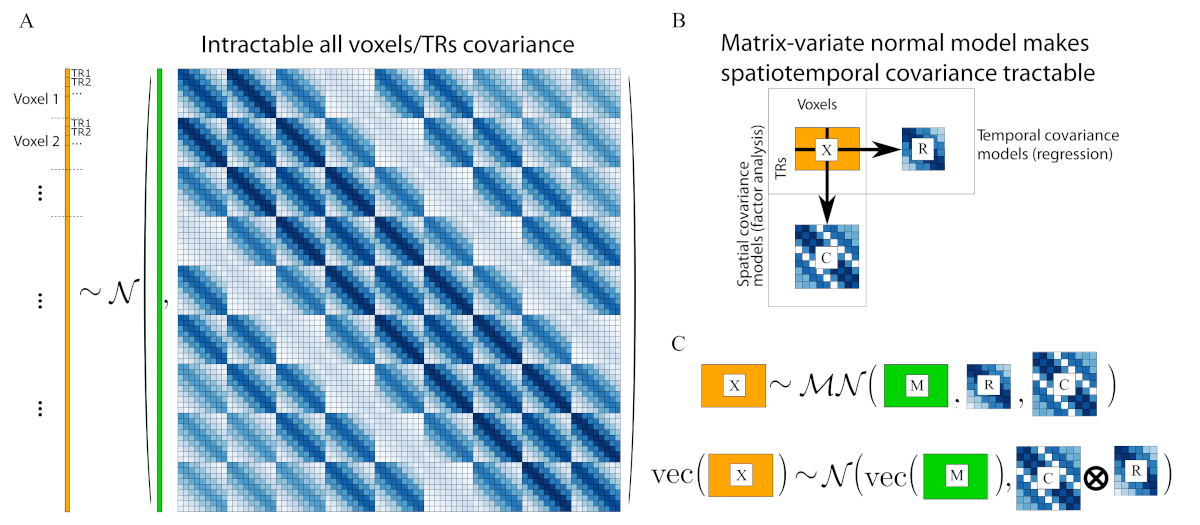# Rapid prototyping of fMRI models with brainiak.matnormal¶

Michael Shvartsman m.shvartsman.work@gmail.com

# Annotated Bibliography¶

Shvartsman, M., Sundaram, N., Aoi, M., Charles, A., Willke, T. L., & Cohen, J. D. (2018). Matrix-normal models for fMRI analysis. International Conference on Artificial Intelligence and Statistics, AISTATS 2018, 1914–1923. Extended version available at link Describes how to formulate a number of common fMRI analysis methods available in BrainIAK as matrix-normal models, and shows some benefits of this formulation.

Cai, M. B., Shvartsman, M., Wu, A., Zhang, H., & Zhu, X. (2020). Incorporating structured assumptions with probabilistic graphical models in fMRI data analysis. Neuropsychologia, 144, 1–23. link Provides an alternate framing of the matrix normal model focusing on the modeling of structured residuals.

Magnus, J. R., & Neudecker, H. (1988). Matrix differential calculus with applications in statistics and econometrics. This is the standard reference for matrix calculus. A summary of some important identities may also be found on Wikipedia at link.

Katanoda, K., Matsuda, Y., & Sugishita, M. (2002). A spatio-temporal regression model for the analysis of functional MRI data. NeuroImage, 17(3), 1415–1428. link Example of a regression model for fMRI with separable residuals.

Hartvig, N. V. (2002). A stochastic geometry model for functional magnetic resonance images. Scandinavian Journal of Statistics, 29(3), 333–353. link Example of a separable residual covariance to a spatial activation model for fMRI data.

Kia, S. M., Beckmann, C. F., & Marquand, A. F. (2018). Scalable multi-task gaussian process tensor regression for normative modeling of structured variation in neuroimaging data. Retrieved from link Example of using tensor regression models for analyzing fMRI data.

## Overview: Understanding kronecker-separability¶

Unlike many of the other tools in brainiak, the brainiak.matnormal package is only a little bit about specific methods and a lot about letting you try new ideas and method variants quickly. If the variants are useful, they can be sped up and made neater for broader consumption. To understand the idea behind matrix-normal or kronecker-separable models, consider the following figure:Matrix normal models simultaneously model spatial and temporal residuals. [A]: a schematic view of a vectorized data matrix, where each voxel's time series is vertically concatenated (in orange), and the covariance of every voxel at every timepoint with every other voxel at every other timepoint is modeled. Modeling all of these elements independently is intractable, and some structure needs to be imposed -- in this case, kronecker-separable structure. [B]: the un-vectorized data matrix (orange rectangle), and its spatial and temporal covariances on the right and bottom. [C]: A matrix-normal distribution with the mean M and row/column covariances R, C is equivalent to the large structure in [A], but can be much more tractable to estimate. Figure and caption reused under CC-BY-NC-ND from doi:10.1016/j.neuropsychologia.2020.107500.

## Example 1: Matrix-normal (separable-covariance) regression¶

To understand how simple it is to prototype new matrix-normal models, consider first simple linear regression:

$$Y \sim \mathcal{MN}(XB, I, I),$$

where $Y$ is a centered TRs by voxels matrix of brain data, $X$ is a TRs by conditions design matrix, and $B$ is a conditions by voxels coefficients matrix. Notated this way, the model is the conventional massively-univariate GLM: the activation at every voxel and every timepoint is a linear combination (aka weighted sum) of the condition coding, weighted by the coefficients -- or equivalently a weighted sum of the coefficients, with the condition codes as weights. We can generate a simple dataset and show how simple it is to fit such a model by maximum likelihood using automatically computed gradients.

In practice, a simple model like this could just as easily be fit using more standard tools. More interesting is if we (reasonably) assume that the noise in our data is structured, and we want to model that structure rather than try to prewhiten it, for example:

$$\sigma_v^{-1} \sim \mathcal{InvGamma}(\alpha, \beta)\\ Y \sim \mathcal{MN}(XB, \Sigma_{AR1},\mathrm{diag}(\sigma_v)),$$

where $\Sigma_{AR1}$ is a structured covariance matrix with AR(1) structure, and the spatial covariance is diagonal (independent) but with varying scales for each voxel and we use an inverse-gamma prior to regularize them. Estimating this model is very similar:

brainiak.matnormal provides a convenience wrapper for such regression models (as MatnormalRegression).

The covariance-modeling approach should work better than either ignoring covariance structure in the data, or pre-whitening by dividing out the correlations. So we now compare to both of those approaches on the same synthetic dataset.